• FN算法
千次阅读
2020-10-29 16:20:22

贪心算法FN具体步骤如下所述：

（1）去掉网络中所有的边，网络的每个节点都单独作为一个社区；

（2）网络中的每个连通部分作为一个社区，将还未加入网络的边分别重新加回网络，如果加入网络的边连接了两个不同的社区，即合并了两个社区，则计算形成的新的社区划分的模块度的增量。选择使模块度增长最大或者减小最少的两个社区进行合并；

（3）如果网络的社区数大于1，则返回步骤 （2） 继续迭代，否则转到步骤 （4）；

（4）遍历每种社区划分对应的模块度的值，选取模块度最大的社区划分作为网络的最优划分。

import networkx as nx
import matplotlib.pyplot as plt
import sys
sys.path.append('./')

class FN:
def __init__(self,G):
self.G = G
self.G_copy = G.copy()
self.edges = [n for n in self.G.edges]                  #把网络中的所有边保存在edges中
#print(self.edges)
self.G.remove_edges_from(self.edges)                    #将网络G中的所有图删除
self.temp_q = {}                                        #存放每一轮的加入边后，Q的变化值、社区划分现状{q差值：community}
self.temp_e = {}                                        #存放每一轮加入边后，Q的变化值、加入边的两个节点{q差值：（node1，node2）}
self.max_Q = self.cal_Q([list(c) for c in list(nx.connected_components(self.G))],self.G_copy) #计算合并社区后新的模块度
self.op_Q = {}                                                 #存放每轮加入边后的Q值，和社区划分情况
#print([list(c) for c in list(nx.connected_components(self.G))])
def run(self):
while len(self.G.edges) != len(self.G_copy.edges):        #判断是否将所有边连接回去
for e in self.edges:                                  #将边列表的每条边连接，并计算一下Q差值
self.merge_community(e,e)

#self.max_Q=max(self.temp_e)
self.max_Q = self.cal_Q(self.temp_q[max(self.temp_q)],self.G_copy)                  #计算合并社区后的模块度

self.edges.remove(tuple(self.temp_e[max(self.temp_e)]))                             #从边的集合中将加入网络的边删除

self.op_Q[self.max_Q]=self.temp_q[max(self.temp_q)]                                 #将每次选中边加入网络后的Q，与社区划分状态保存
self.temp_e.clear()                                                                 #将这一轮尝试边留下的临时信息删除，以免影响下一轮
self.temp_q.clear()

def merge_community(self,n1,n2):                                               #加入边，并记录这条边加入后Q的变化量

components = [list(c) for c in list(nx.connected_components(self.G))]
#print(components)
#print(self.cal_Q(components,self.G_copy))
self.temp_q[self.cal_Q(components,self.G_copy)-self.max_Q]=components
#print(self.temp_q)
self.temp_e[self.cal_Q(components,self.G_copy)-self.max_Q]=[n1,n2]
#print(self.temp_e)
self.G.remove_edge(n1,n2)

def cal_Q(self,partition,G):                      #计算Q
m = len(G.edges(None, False))                 #如果为真，则返回3元组（u、v、ddict）中的边缘属性dict。如果为false，则返回2元组（u，v）
#print(G.edges(None,False))
#print("=======6666666")
a = []
e = []
for community in partition:                   #把每一个联通子图拿出来
t = 0.0
for node in community:                    #找出联通子图的每一个顶点
t += len([x for x in G.neighbors(node)])            #G.neighbors(node)找node节点的邻接节点
a.append(t/(2*m))
#             self.zidian[t/(2*m)]=community
for community in partition:
t = 0.0
for i in range(len(community)):
for j in range(len(community)):
if(G.has_edge(community[i], community[j])):
t += 1.0
e.append(t/(2*m))

q = 0.0
for ei,ai in zip(e,a):
q += (ei - ai**2)
return q

num = 0
nodegroup = {}
for partition in self.op_Q[max(self.op_Q)]:
for node in partition:
nodegroup[node] = {'group':num}
num = num + 1
nx.set_node_attributes(self.G_copy, nodegroup)

def setColor():
color_map=[]
color=['red','green','yellow','pink','blue']
for i in a.G_copy.nodes.data():
color_map.append(color[i["group"]])
return color_map

a = FN(G)
a.run()
#print(a.temp_e)
print("============================执行结果========================")
print("MAX_Q:")
print(max(a.op_Q))
print("最优划分:")
print(a.op_Q[max(a.op_Q)])
#ga = nx.to_numpy_array(G)
nx.draw(G,with_labels=True,node_color=setColor())
plt.show()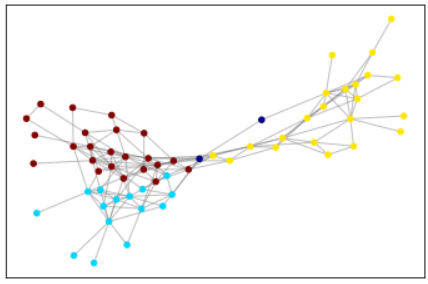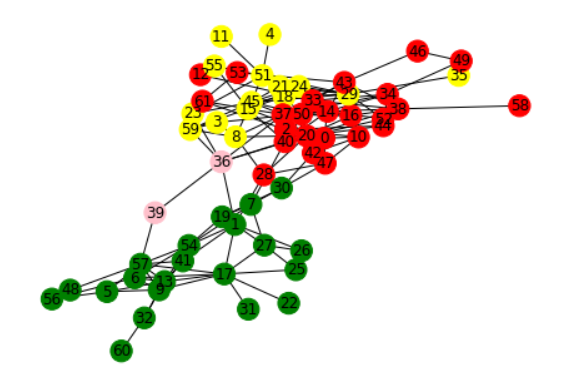实现结果似乎一致，但运行花费时间太长。python
更多相关内容
• RRT、RRT* 和 RRT*FN 算法的 MATLAB 实现。 什么是 RRT、RRT* 和 RRT*FN RRT（Rapidly-Exploring Random Tree）是一种基于采样的算法，用于解决路径规划问题。如果 RRT 的时间趋于无穷大，RRT 提供了可行的解决方案...
• 无第三方库的高效FN算法python实现。

### 效率非常高的FN算法Python实现

前几期推送介绍了一些非重叠社区发现算法（GN算法、FN算法），比较适用于小网络社区检测。FN算法计算效率相较于GN算法有一定提升，且社区划分效果不错。以下是GN、FN算法具体介绍和对比：

社区发现之FN算法Python实现中，需要多次计算合并两个社区的增益 Δ Q \Delta{Q} ，但是 Δ Q \Delta{Q} 的计算方式的不同也会影响算法最终的一个计算效率，因此本文针对 Δ Q \Delta{Q} 的计算方式进行了一些优化，相较于社区发现之FN算法Python实现中的计算效率有很大提升。以下是优化前后计算时间在不同网络下的对比（第一列是测试用例及网络规模，第二、三列为Q和计算时间，FN：优化前，Fast-N：优化后）。Newman, M. E. J. ,2004. Fast algorithm for detecting community structure in networks. phys rev e stat nonlin soft matter phys, 69, 066133.

去看原文

## 基本概念

在社区中有几个关键概念需要先进行说明，以下是FN社区合并的一个示意图：FN算法是一种层次聚类算法。起初每个节点都是一个类。每次合并让Q值增加（即 Δ Q \Delta{Q} ）最大的一对节点，重复这个过程，直到所有节点都在一个社区为止。在这个合并的过程中，选择Q值（社区发现评估指标）最大的作为最终划分结果。

Δ Q = 2 ( e i j − a i a j ) \Delta{Q}=2(e_{ij}-a_ia_j)
其中， e i j e_{ij} 表示连接社区 i i 和社区 j j 的边的比例； a i a_i 表示连接到社区 i i 的所有末端节点比例， a i = ∑ j e i j a_i=\sum_j{e_{ij}}

那么上图各个社区的几个关键量如下图（注意，为书写方便，以下的量没有除以网络边的总数），并给出这些量合并两个社区的动态更新公式：同时， e i i e_{ii} 这个量可以用来计算模块度Q。因此，我们需要将上述三个量在算法计算过程中进行保存，并在合并社区后进行动态更新，就无需采用遍历的方式重新计算这些量。

## 优化实现

在这里，为了方便设计数据结构来存储中间变量，我们不使用Python任何第三方库进行实现。

# -*- coding: utf-8 -*-
# @Author: 武辛
# @Email: geo_data_analysis@163.com
# @Note: 如有疑问，可加微信"wxid-3ccc"
​
import sys, copy, time
​
def FN():
​
max_Q = float("-inf"); partition = None
start_time = time.time()
while len(network.Communities) > 0:
print("left %d communities need to merge, waiting..." % len(network.Communities))
det_Q = float("-inf")
​
if community_i == community_j: continue
​
# 计算两个community的det_Q
cur_Q = cal_det_Q(network, community_i, community_j)
​
# 找到合并两个community Q值增加最大的进行合并
if cur_Q > det_Q:
det_Q = cur_Q
if max_link is None: break
​
# 合并两个community，将社区j合并到社区i中
merge(network, community_i, community_j)
​
# 合并社区j后，更新社区i的e_ii,a_i,e_ij信息
update_community_info(network, community_i, community_j)
​
# 删除边ij
​
# 计算合并社区ij后的模块度
cur_Q = cal_Q(network)
if cur_Q > max_Q:
max_Q = cur_Q
partition = copy.deepcopy(list(network.Communities.values()))
​
t2 = time.time()
print("Find %d communities after %.3f seconds, the maximal Q is %.3f" % (len(partition), t2 - start_time, max_Q))
​
if __name__ == '__main__':
FN()

load the network...
left 22 communities need to merge, waiting...
left 21 communities need to merge, waiting...
left 20 communities need to merge, waiting...
left 19 communities need to merge, waiting...
left 18 communities need to merge, waiting...
left 17 communities need to merge, waiting...
left 16 communities need to merge, waiting...
left 15 communities need to merge, waiting...
left 14 communities need to merge, waiting...
left 13 communities need to merge, waiting...
left 12 communities need to merge, waiting...
left 11 communities need to merge, waiting...
left 10 communities need to merge, waiting...
left 9 communities need to merge, waiting...
left 8 communities need to merge, waiting...
left 7 communities need to merge, waiting...
left 6 communities need to merge, waiting...
left 5 communities need to merge, waiting...
left 4 communities need to merge, waiting...
left 3 communities need to merge, waiting...
left 2 communities need to merge, waiting...
left 1 communities need to merge, waiting...
Find 3 communities after 0.006 seconds, the maximal Q is 0.528


## 对比实验

为了验证该算法的效率，我们采用了几个网络（自定义测试网络、dolphins、football、collaboration）来进行对比实验，以下是这几个网络的详细信息：collaboration网络（9875个节点，25973条边）用优化后的FN算法（见）需要989.2秒出结果，先前的实现方式需要12小时以上（时间太长，没有跑完）。可以看出，通过动态更新的方式可以很大程度上提升FN的计算效率。

去看原文

去看原文

去看原文

更多内容，请关注地学分析与算法。展开全文社区发现 FN算法
• 社区发现FN算法的Python实现，并与GN算法在结果优劣以及计算效率方面进行对比。

### 社区发现FN算法Python实现

​2004年，Newman在GN(Girvan and Newman, 2002)算法的基础上，提出了另外一种快速检测社区的算法，称为FN算法。该算法能得到和GN算法相似的结构，但是时间复杂度更低，GN算法的时间复杂度为 O ( m 2 n ) O(m^2n) ，FN算法的时间复杂度为 O ( ( m + n ) n ) O((m+n)n) ，其中， m m 是边的数量， n n 是节点的数量。此处给出FN算法的Python实现，并给出对比实验以及社区发现的三种评价指标。Newman, M. E. J. ,2004. Fast algorithm for detecting community structure in networks. phys rev e stat nonlin soft matter phys, 69, 066133.

去看原文

## 算法原理

FN算法是一种层次聚类算法。起初每个节点都是一个类。每次合并让Q值增加（即 Δ Q \Delta{Q} ）最大的一对节点，重复这个过程，直到所有节点都在一个社区为止。在这个合并的过程中，选择Q值（社区发现评估指标）最大的作为最终划分结果。

Δ Q = 2 ( e i j − a i a j ) \Delta{Q}=2(e_{ij}-a_ia_j)
其中， e i j e_{ij} 表示连接社区 i i 和社区 j j 的边的比例； a i a_i 表示连接到社区 i i 的所有末端节点比例， a i = ∑ j e i j a_i=\sum_j{e_{ij}} 。以下是一个合并的结构图，从下往上进行合并。## 评价指标

社区发现的评估指标主要有三个：互信息和标准化互信息（Normalized Mutual Information，NMI指数）、调整兰德指数（Adjusted Rand Index，ARI指数）、模块度Q（modularity Q）。

当无法获取真实社区划分结果时，可以采用模块度Q来评价。Modularity用于评判社区划分结果的优劣。模块度越大则表明社区划分效果越好，其范围在 [ − 0.5 , 1 ) [-0.5,1) ，论文(Newman, 2003)表示当Q值在0.3~0.7之间时，说明聚类的效果很好。

Q = ∑ i = 1 n ( e i i − a i 2 ) Q=\sum_{i=1}^{n}(e_{ii}-a_i^2)
其中 e i j = ∑ v w A v w 2 m e_{ij}=\sum_{vw}\frac{A_{vw}}{2m} a i = k i 2 m = ∑ j e i j a_i=\frac{k_i}{2m}=\sum_je_{ij}
m m 表示边的数量， e i j e_{ij} 表示一个节点在社区 i i 内，另一个节点在社区 j j 内的边的比例。 e i i e_{ii} 表示在社区 i i 内所有的边与整个网络所有的边的一个比值（一个社区内部的度比上整个网络的度），而 a i a_{i} 则表示i社区内的节点的度（包含了一点在社区 i i 内一点在社区 i i 外的边的度）占整个网络的度比值。

可将模块度用矩阵形式表示，即
Q = 1 2 m T r ( S T B S ) Q=\frac{1}{2m}Tr(S^TBS)
其中， B i j = A i j − k i k j 2 m B_{ij}=A_{ij}-\frac{k_ik_j}{2m} k i k_i 代表的是节点 i i 的度， A i j A_{ij} 为邻接矩阵; S S 为每个节点所属社区的one-hot表示， S i r = 1 S_{ir}=1 表示第 i i 个节点属于第 r r 社区。

当已知真实社区划分结果时，可采用NMI指数和ARI指数进行评价。
1.NMI指数
如果结果越相似NMI值应接近1；结果很差则NMI值接近0。
N M I ( X , Y ) = 2 M I ( X , Y ) H ( X ) + H ( Y ) NMI(X,Y)=\frac{2MI(X,Y)}{H(X)+H(Y)}
其中， M I ( X , Y ) = ∑ i = 1 ∣ X ∣ ∑ j = 1 ∣ Y ∣ P ( i , j ) l o g ( P ( i , j ) P ( i ) P ′ ( j ) ) MI(X,Y)=\sum_{i=1}^{|X|}\sum_{j=1}^{|Y|}P(i,j)log(\frac{P(i,j)}{P(i)P'(j)}) H ( X ) = − ∑ i = 1 ∣ X ∣ P ( i ) l o g ( P ( i ) ) H(X)=-\sum_{i=1}^{|X|}P(i)log(P(i)) H ( Y ) = − ∑ j = 1 ∣ Y ∣ P ′ ( j ) l o g ( P ′ ( j ) ) H(Y)=-\sum_{j=1}^{|Y|}P'(j)log(P'(j)) X ， Y X，Y 是划分类别唯一标签和真实类别唯一标签。

以下将用一个例子来介绍如何计算。
输出的划分结果：A=[1 2 1 1 1 1 1 2 2 2 2 3 1 1 3 3 3]
真实的划分结果：B=[1 1 1 1 1 1 2 2 2 2 2 2 3 3 3 3 3]

那么 X = u n i q u e ( A ) = [ 1 , 2 , 3 ] ， Y = u n i q u e ( B ) = [ 1 , 2 , 3 ] X=unique(A)=[1,2,3]，Y=unique(B)=[1,2,3]
P ( i , j ) P(i,j) 表示同时属于社区 i i 和社区 j j 的节点的联合概率， P ( i , j ) = ∣ X i ⋂ Y j ∣ N P(i,j)=\frac{|X_i\bigcap{Y_j}|}{N} , N N 为节点数, i ∈ X , j ∈ Y i\in{X},j\in{Y}
P ( i ) , P ( j ) P(i),P(j) 分别为类别 i , j i,j 的概率分布， P ( i ) = ∣ X i ∣ N , P ′ ( j ) = ∣ Y j ∣ N P(i)=\frac{|X_i|}{N},P'(j)=\frac{|Y_j|}{N}
H ( X ) , H ( Y ) H(X),H(Y) 分别为 X , Y X,Y 的信息熵。
所以 P ( X ) = [ 8 / 17 , 5 / 17 , 4 / 17 ] P(X)=[8/17,5/17,4/17] P ( Y ) = [ 6 / 17 , 6 / 17 , 5 / 17 ] P(Y)=[6/17,6/17,5/17]
P ( X , Y ) = [ 5 / 17 1 / 17 2 / 17 1 / 17 4 / 17 0 0 1 / 17 3 / 17 ] P(X,Y)=\begin{bmatrix} 5/17 & 1/17 & 2/17 \\ 1/17 & 4/17 & 0 \\ 0 & 1/17 & 3/17 \\ \end{bmatrix}
因此， M I ( X , Y ) = s u m ( P ( X , Y ) ∗ l o g ( P ( X , Y ) / ( P ( X ) T P ( Y ) ) ) ) MI(X,Y)=sum(P(X,Y) * log(P(X,Y)/(P(X)^TP(Y)))) H ( X ) = − P ( X ) l o g ( P ( X ) T ) H(X)=-P(X)log(P(X)^T) H ( Y ) = − P ( Y ) l o g ( P ( Y ) T ) H(Y)=-P(Y)log(P(Y)^T) ，则 N M I ( X , Y ) = 0.3646 NMI(X,Y)=0.3646

2.ARI指数
兰德指数（RI指数）是两种划分 X , Y X,Y 中顶点对正确分类的数量（顶点对在同一个社团中或者在不同的社团中）与总的顶点对的数量的比值，可以使用下式表示：
R I ( X , Y ) = a 00 + a 11 a 00 + a 01 + a 10 + a 11 = a 00 + a 11 C 2 n RI(X,Y)=\frac{a_{00}+a_{11}}{a_{00}+a_{01}+a_{10}+a_{11}}=\frac{a_{00}+a_{11}}{C_2^n}
其中， a 00 a_{00} 表示在真实社团划分与实验得到的社团划分里都不属于同一社团的点对数目； a 11 a_{11} 表示在真实社团划分与实验得到的社团划分里都属于同一社团的点对数目； C 2 n C_2^n 指可以组成的总顶点对对数。 R I RI 取值范围为 [ 0 , 1 ] [0,1] ，值越大意味着两种划分结果越吻合。然而 R I RI 会存在区分度不高的情况。因此为了提高区分度，提出了ARI指数：
A R I = R I − E ( R I ) m a x ( R I ) − E ( R I ) ARI=\frac{RI-E(RI)}{max(RI)-E(RI)}
A R I ARI 取值范围为 [ − 1 , 1 ] [−1,1] ，值越大意味着两种划分结果越吻合。从广义的角度来讲，ARI衡量的是两个数据分布的吻合程度。

## 结果对比

为了验证FN算法的结果质量和计算效率，采用了3个不同的测试案例来和GN算法进行对比实验。

自定义网络dolphinsfootball
节点数量2262115
边数量37159613

以下是实验结果（football网络有真实划分结果，分割线|前的为GN算法，后为FN算法，即GN | FN）：

QNMIARI计算时间(s)
自定义网络0.528|0.528//0.024|0.02
dolphins0.519|0.495//0.67|0.46
football0.60|0.550.88|0.700.78|0.4710.1|8.8

可以看出，FN算法的结果质量稍逊于GN算法，但是计算效率更高。

以下是dolphins网络中两个算法结果的可视化：
FN算法结果（4个社区）：GN算法结果（5个社区）：原文有源码，去看原文

## 一种高效实现

更多内容，请关注地学分析与算法。展开全文社区发现 GN算法 FN算法 社区发现评价指标
• ## 社区发现算法——GN算法与FN算法

千次阅读 热门讨论 2022-02-04 10:28:28
GN算法算法的具体内容请参考Finding and evaluating community structure in networks（Newman and Girvan）。 重要概念 边介数（betweenness）：网络中任意两个节点通过此边的最短路径的数目。 GN算法的思想： ...

# GN算法

重要概念

边介数（betweenness）：网络中任意两个节点通过此边的最短路径的数目。

GN算法的思想：

在一个网络之中，通过社区内部的边的最短路径相对较少，而通过社区之间的边的最短路径的数目则相对较多。GN算法是一个基于删除边的算法，本质是基于聚类中的分裂思想，在原理上是使用边介数作为相似度的度量方法。在GN算法中，每次都会选择边介数高的边删除，进而网络分裂速度远快于随机删除边时的网络分裂。

GN算法的步骤如下：
（1）计算每一条边的边介数；
（2）删除边界数最大的边；
（3）重新计算网络中剩下的边的边阶数；
（4）重复(3)和(4)步骤，直到网络中的任一顶点作为一个社区为止。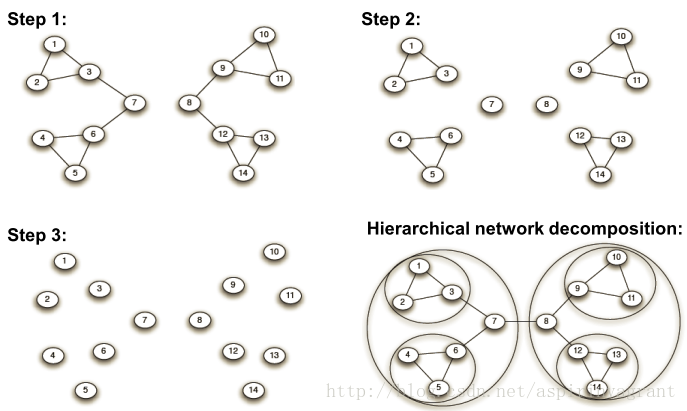GN算法计算边界数的时间复杂度为 O(m*n)，总时间复杂度在m条边和n个节点的网络下为 O(m^2 *n)。
GN算法的缺陷：
（1）不知道最后会有多少个社区；
（2）在计算边介数的时候可能会有很对重复计算最短路径的情况，时间复杂度太高；
（3）GN算法不能判断算法终止位置。
为了解决这些问题，Newman引入了模块度Q的概念，它用来一个评价社区结构划分的质量。网络中的社区结构之间的边数并不是绝对数量上的少，而是应该比期望的边数要少。

模块度

模块度介绍

设Avw为网络的邻接矩阵的一个元素，定义为：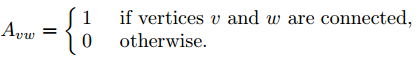假设cv和cw分别表示点v和点w所在的两个社区，社区内部的边数和网络中总边数的比例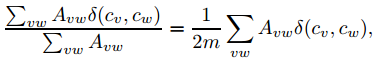函数δ(cv,cw)的取值定义为：如果v和w在一个社区，即cv=cw，则为 1，否则为 0。m 为网络中边的总数。

模块度的大小定义为社区内部的总边数和网络中总边数的比例减去一个期望值，该期望值是将网络设定为随机网络时同样的社区分配所形成的社区内部的总边数和网络中总边数的比例的大小，于是模块度Q为：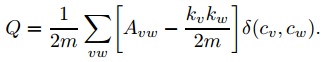其中kv表示点v的度。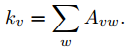设eij表示社区I和社区J之间的连接边和与总边数的比例，ai表示社区i内部的点所关联的所有的边的数目与总边数的比例。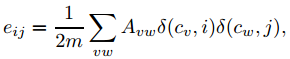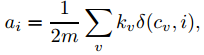为了简化Q的计算，假设网络已经划分成n个社区，这个时候就有一个 n维矩阵，Q 的计算可以变成：

eii表示的是节点全在社区i内部中的边所占的比例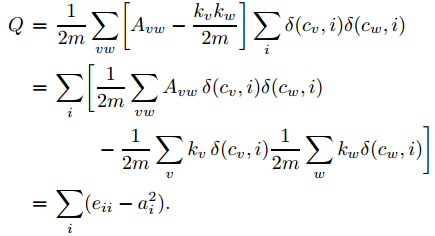模块度的物理意义是，网络中连接两个同种类型结点的边(即社区内部的边)的比例减去在同样的社区结构”下任意连接节点的边的比例的期望值。如果社区内部边的比例不大于任意连接时的期望值,则有Q=0。Q的上限为1,而Q越接近于这个值,就说明社区结构越明显。实际网络中，该值通常位于0.3-0.7之间。

引入模块度后代码如下：

1. 计算当前网络的边介数和模块度Q值，并存储模块度Q值和当前网络中社团分割情况
2. 除去边介数最高的边；
3. 计算当前网络的模块度Q值，如果此Q值比原来的大，则将现在的Q值和网络中社团分割情况存储更新，否则，进行下一次网络分割；
4. 所有边分割完毕，返回当前的模块度Q值和community分割情况。

数据集为Karate数据集：
Zachary空手道俱乐部成员关系网络是复杂网络、社会学分析等领域中最常用的一个小型检测网络之一。从1970到1972年，Zachary观察了美国一所大学空手道俱乐部成员间的社会关系，并构造出了34个成员，78条成员关系的社会关系网。两个成员经常一起出现在俱乐部活动之外的其他场合，就认为两个成员间有边。该俱乐部因为主管(节点34)与教练(节点1)之间的争执而分裂成2个各自为核心的小俱乐部。

python代码如下：

class GN:
def __init__(self, G):
self._G = G
self._G_cloned = cloned_graph(G)
# 初始划分情况为所有节点为一个社区
self._partition = [[n for n in G.nodes()]]
self._max_Q = 0.0

def execute(self):
while len(self._G.edges()) != 0:
# 1.计算每一条边的边介数
# nx.edge_betweenness返回边介数字典，items返回可遍历的(键, 值) 元组数组。这里每个item是((vi,vj), edge_betweenness))
# 因此我们取的item最大的item，再取该最小item的，为我们要删除的两个点(即要删除的边)
edge = max(nx.edge_betweenness_centrality(self._G).items(),
key=lambda item: item)
# 2. 移去边介数最大的边
self._G.remove_edge(edge, edge)
# 获得移去边后的子连通图
components = [list(c)
for c in list(nx.connected_components(self._G))]
if len(components) != len(self._partition):
# 3. 计算Q值
cur_Q = cal_Q(components, self._G_cloned)
if cur_Q > self._max_Q:
self._max_Q = cur_Q
self._partition = components
print(self._max_Q)
print(self._partition)
return self._partition


结果如下：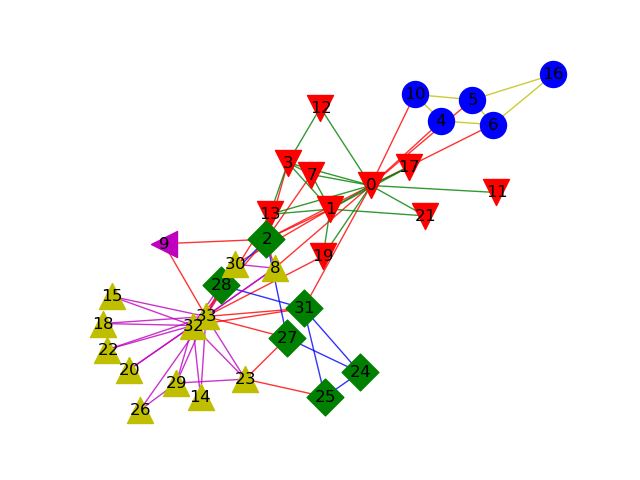Q值：0.40129848783694944
算法执行时间0.08202219009399414

# Newman快速算法 (FN算法)

GN算法通过模块度可以准确的划分网络，但它只适用于中小型规模的网络(时间复杂度高)。Newman提出一种基于贪心的快速社区发现算法，算法的基本思想是：首先将网络中的每个顶点设为一个单独社区，每次迭代选择产生最大Q值两个社团合并，直至整个网络融合成一个社团。整个过程是自底向上的过程，且这个过程最终得到一个树图，即树的叶子节点表示网络中的顶点，树的每一层切分对应着网络的某个具体划分，从树图的所有层次划分中选择模块度值最大的划分作为网络的有效划分。该算法的总体时间复杂度为Ｏ（ｍ（ｍ＋ｎ））

设网络有n个节点，m条边，每一步合并对应的社区数目为r，组成一个r*r矩阵e，矩阵元素eij表示社区i中的节点与社区j中节点之间连边的数目在网络总变数的百分比。

主要步骤：

（1） 初始化网络，开始网络有n 个社区，初始化的eij和ai为：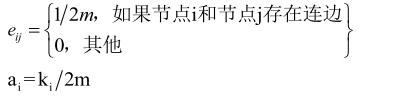（2）依次按照∆Q的最大或者最小的方向进行合并有边相连的社区对，并计算合并后的模块度增量∆Q：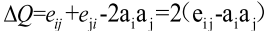（3）合并社区对以后修改对社区对称矩阵e 和社区i和j对应的行列；

（4）重复执行步骤（2）和（3），不断合并社区，直至整个网络合并成一个社区为止。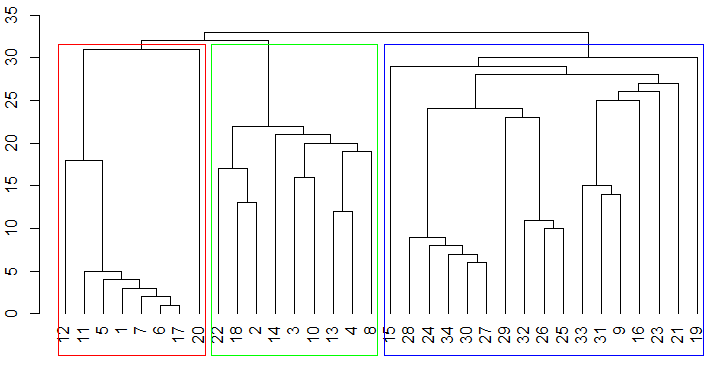python代码如下：

class FastNewman:
def __init__(self, path):
# G = nx.read_gml('dolphins.gml')
self.A = nx.to_numpy_array(self.G)  # 邻接矩阵
self.num_node = len(self.A)  # 点数
self.num_edge = sum(sum(self.A))  # 边数
self.c = {}  # 记录所有Q值对应的社团分布

# def merge_community(self, iter_num, detaQ, e, b):
#     # 一起合并容易出bug  查询的结果I在遍历过程中 可能在已经前面某次作为J被合并了
#     # 比如某次是[ 3, 11] [11, 54] 第一轮迭代中11被合并 第二轮54合并到旧的11中  会导致后面被删除 导致节点消失  需要将54合并到现在11所在位置  比较麻烦 不如一个个合并
#     b_num = sum([len(i) for i in b])
#     det_max = np.amax(detaQ)
#
#     (I, J) = np.where(detaQ == det_max)
#     print((I, J) )
#     # 由于先遍历的I I中可能有多个相同值  所以合并时候因应该将J合并到I中
#     # 如果将I合并到J中 后续删除删不到
#     for m in range(len(I)):
#         # 确保J还未被合并
#         if J.tolist().index(J[m]) == m:
#             # 将第J合并到I 然后将J清零
#             e[I[m], :] = e[J[m], :] + e[I[m], :]
#             e[J[m], :] = 0
#             e[:, I[m]] = e[:, J[m]] + e[:, I[m]]
#             e[:, J[m]] = 0
#             b[I[m]] = b[I[m]] + b[J[m]]
#
#     e = np.delete(e, J, axis=0)
#     e = np.delete(e, J, axis=1)
#     J = sorted(list(set(J)), reverse=True)
#     for j in J:
#         b.remove(b[j])  # 删除第J组社团，（都合并到I组中了）
#     b_num2 = sum([len(i) for i in b])
#     if b_num2 != b_num:
#         print("111")
#     self.c[iter_num] = b.copy()
#     return e, b

def merge_community(self, iter_num, detaQ, e, b):
# 一个个合并
(I, J) = np.where(detaQ == np.amax(detaQ))
# 由于先遍历的I I中可能有多个相同值  所以合并时候因应该将J合并到I中
# 如果将I合并到J中 后续删除删不到
e[I, :] = e[J, :] + e[I, :]
e[J, :] = 0
e[:, I] = e[:, J] + e[:, I]
e[:, J] = 0
b[I] = b[I] + b[J]

e = np.delete(e, J, axis=0)
e = np.delete(e, J, axis=1)
b.remove(b[J])  # 删除第J组社团，（都合并到I组中了）
self.c[iter_num] = b.copy()
return e, b

def Run_FN(self):
e = self.A / self.num_edge  # 社区i,j连边数量占总的边的比例
a = np.sum(e, axis=0)  # e的列和，表示与社区i中节点相连的边占总边数的比例
b = [[i] for i in range(self.num_node)]  # 本轮迭代的社团分布
Q = []
iter_num = 0
while len(e) > 1:
num_com = len(e)
detaQ = -np.power(10, 9) * np.ones((self.num_node, self.num_node))  # detaQ可能为负数，初始设为负无穷
for i in range(num_com - 1):
for j in range(i + 1, num_com):
if e[i, j] != 0:
detaQ[i, j] = 2 * (e[i, j] - a[i] * a[j])
if np.sum(detaQ + np.power(10, 9)) == 0:
break

e, b = self.merge_community(iter_num, detaQ, e, b)

a = np.sum(e, axis=0)
# 计算Q值
Qt = 0.0
for n in range(len(e)):
Qt += e[n, n] - a[n] * a[n]
Q.append(Qt)
iter_num += 1
max_Q, community = self.get_community(Q)
return max_Q, community

def get_community(self, Q):
max_k = np.argmax(Q)
community = self.c[max_k]
return Q[max_k], community


运行结果如下：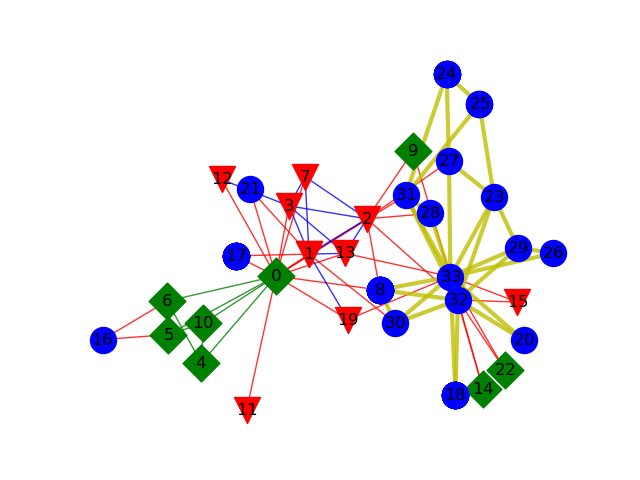Q值：0.3806706114398422
算法执行时间0.00400090217590332
可以看到与GN算法相比，GN算法模块度略微下降，但执行时间快了很多。

代码与数据集下载

展开全文机器学习 人工智能
• ## 社区发现FN算法

热门讨论 2013-07-23 13:57:33
Newman的文章Fast algorithm for detecting community structure in networks对应的算法，有详细的说明，并附有例子数据。
• rrt_toolbox：MATLAB的RRT，RRT *，RRT * FN算法
• Newman快速算法，是一种凝聚算法，基于python的复杂网络库--Networkx实现，有源数据和网络可视化呈现
•matlab
• ## 基于Networkx的社区发现算法（FN）

千次阅读 热门讨论 2019-11-15 15:38:29
基于Networkx的FN社区发现算法 ...2、找到一篇FN算法的讲解，但是用Matlab实现的，因为不方便实现网络可视化，所以转成了python版，以下是原文链接：FN算法详解. （其中detaQ=(eji+eij-2aiaj)=2(...python Networkx
• 近期想对社区发现领域进行一下简单研究，看到一篇不错的文章，文章是根据国防科大骆志刚教授的论文《复杂网络社团发现算法研究新进展》整理的，主要是对社区发现的一些算法进行简单分析。 一、基于模块度优化的...社区发现
• 09498664GN_FN.zip,GN_FN,说明.doc,G_N&F_N,0.5_4.gra,in94.mat,0.5_1.gra,in85.mat,0.9_5.gra,Accuracy1.m,in63.mat,0.7_3.gra,in10_1.mat,in75.mat,in65_1.mat,0.2_1.gra,0.6_4.gra,main2.asv,0.9_2.gra,0.4_1.gra...
• 包含了国密SM3摘要算法的java实现，可以实现基本的生成消息摘要的功能。
• 算法设计与分析-模拟试题(1).docx算法设计与分析-模拟试题(1).docx算法设计与分析-模拟试题(1).docx算法设计与分析-模拟试题(1).docx
• 基于FN空间神经网络二值图像增强算法研究.pdf
• 利用循环队列编写求k阶斐波那契序列中第n+1项fn算法 ##记录一下自己做题时的历程 错误示例： SqQueue Q; Q.base=(ElemType )malloc(ksizeof(ElemType)); Q.maxSize=k; Q.front=Q.rear=0; if(k<1||Q.maxSize<...数据结构
• 相关概念 复杂网络：具有自组织、自相似、吸引子、小世界、无标度中部分或全部性质的网络称为复杂网络。 社团结构：网络中的顶点可以分成组,组内顶点间的连接比较稠密,组间顶点的连接比较稀疏。...聚合：FN
• ## 加权GN算法简介

万次阅读 2016-02-28 21:38:31java
• ## 社区发现算法——Louvain 算法

千次阅读 多人点赞 2022-02-08 16:07:40
Louvain 算法 原始论文为：《Fast unfolding of communities in large networks》。 所以又被称为Fast unfolding算法。 Louvain算法是一种基于模块度的社区发现算法。其基本思想是网络中节点尝试遍历所有邻居的社区...机器学习
• 社区挖掘是复杂网络分析中的一项重要工作，目前已提出多种社区挖掘算法，但多数...实验结果表明，该方法能够快速揭示出网络中的社区结构，相比FN算法，具有较高的准确度和模块度，相比GN算法，不需要预先知道社区个数。
• 尽管Python事实上并不是一门纯函数式编程语言，但它本身是一门多范型语言，并给了你足够的自由利用函数式编程的便利。函数式风格有着各种理论与实际上的好处（你可以在Python的文档中找到这个列表）： ...
•贪心算法
• 6 个真实数据集上针对外部指标和内部指标的实验结果均表明，相比于传统的FN算法，NFN算法能发现更高质量的社区． 在参数设置方面，长结构优先策略优于短结构优先策略，且采用k-clique结构作为核心紧耦合结构优于...
• } 大一新生LinYX 最近学了一个新的算法—递归，他发现这个算法可以解决一些高中的数列问题，如果已知f1以及公式fn=a*fn-1+b，求fn很方便。聪明的你也应该已经学会了递归，那就来表现一下吧。 在这里给出一组输入。...
• Fibonacci数列的递推公式为：Fn=Fn-1+Fn-2，其中F1=F2=1。 当n比较大时，Fn也非常大，现在我们想知道，Fn除以10007的余数是多少。 <此题禁止使用数组容器等数据结构> 【输入格式】 输入一行包含一个整数 n。 ...java
• 信道传输函数转换成冲激响应，并且实现LMS算法的自适应均衡
• 使用C++编写的FN算法，使用常用的Dev或者其他的开发软件可以直接解压后运行
• 基于MATLAB的车牌识别算法仿真 [fn,pn,fi]=uigetfile('*.jpg','选择图片'); I=imread([pn fn]);figure,imshow(I);title('原始图像');%显示原始图像 chepailujing=[pn fn] I_bai=I; [PY2,PY1,PX2,PX1]=caitu_fenge(I)...
• 斐波那契数列：Fn = Fn-1 + Fn-2 （ n = 1,2 fib(1) = fib(2) = 1） 练习：使用递归和非递归的方法来求解斐波那契数列的第 n 项 代码如下： # _*_coding:utf-8_*_ def fibnacci(n): if n == 1...